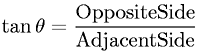Equations > Trigonometry > Trigonometric Definitions > Tangent Definition for a Right Triangle

### Tangent Definition for a Right TriangleLatex Code:

MathML Code:

 $\mathrm{tan}\theta =\frac\mathrm{Opposite}\mathrm{Side}\mathrm{AdjacentSide}$

MathType 5.0: